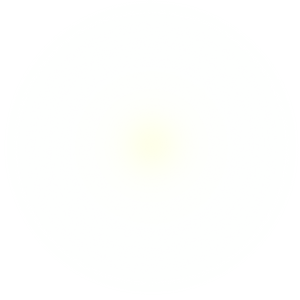What is Infinity?

1. The state or quality of being infinite. Example “the infinity of space”. Synonyms: Endlessness, infinitude, boundlessness, limitlessness.

In Mathematics, infinity is defined as a number greater than any assignable quantity or countable number. ∞.

What if infinity was definable using the number 9. What if Infinity is a value that

1. no matter what it is added to ultimately gives a result equal to the same number it was just added to.

2. no matter what it is multiplied to it ultimately gives the result of itself, that is if infinity is indeed number 9.

Let’s illustrate the first point.

9 + 1 = 10 which if we add 1 + 0 it gives us 1 again.

9 + 2 = 11 same principle, adding the single numbers in our results gives us 2 again.

9 + 3 = 12, back to 3

and so on, we can infinitely go on and we will always get the original number.

9 + 11 = 20, 20 = 2 and 11 = 2 as well in our principle.

9 + 12 = 21, back to 3.

9+ 72 = 81 back to 9.

We can go on and on, ultimately 9 always bring us back to our used single number.

Using this principle, what if infinity could be defined as the ultimate restart. a phenomenon that always brings back to the beginning, to the first value or to itself.

To illustrate that last thought, let’s take a look at our second notion.

9 * 1 = 9

9 * 2 = 18, which according to our first concept is equal to 9 = 1 + 8.

9 * 3 = 27 back to 9.

9 * 15 = 135, (1 + 3 + 5 = 9)

This phenomenon happens infinitely as well.

So if the last number in a base 10 system could be consider as infinite, this could bring a new definition to infinity, instead of being defined as limitless, infinity could be defined as forever returning to base. A little like the earth revolves around the sun and itself.

Earth revolving around itself would be multiplying number 9, while revolving around the sun would be making additions to number 9 but yet always going back to the base.

If we decide to divide 9, we get the best of both worlds. Meaning the results we were getting while doing additions for some numbers as well as the ones we get while multiplying number 9.

For example:

9 ÷ 3 = 3

while

9 ÷ 4 = 2.25 which with our adding all single numbers principle gives us 9 again.

9 ÷ 22 = 0.40909090909 which if if we add up gives us 4 just like 22.

This method of dividing behave quite differently,

if we do 9 ÷ 54 = 0.16666666666 which gives us 7 at the end.

7 defies both of our first tests, the addition and the multiplication.

Is it then safe to say that infinity can only be constructed, it can only add on to what is already there, in that sense, time traveling in the future is very possible while going in the past might be tricky. Although technically if traveling in the future is possible, then living in the past must be a very common.

This last statement can be explained using light and position in space. Light needs to travel to reach destinations, the light from the stars in our sky look to us the way they looked far in the past.

Let’s take the our Godly Sun for example, The light we experience from the sun is about 8 mins after it was emitted. When we laying down tanning on the beach that light caressing our skins was born about 8 mins prior touching us. Now if we add the position element to this, Imagine that I am closer to the Sun than you are, I will experience that same light relatively before you, that puts me in a nearest future compared to you, therefore you are in the past.

If we can travel to the future, we can therefore travel into the past, or better yet if there is a future to travel to, we must be living in its past.

This doesn’t disprove that by definition infinity goes both ways because if infinity have been adding on and multiplying for neons and if infinity is defined by coming back to itself or to whatever is added to it, that means that it had to be an infinite beginning. It had to be a number 9.

It also means that everything either always goes back to its original value or if multiplied by infinity becomes infinity.

9 = ∞.

Maba Ba

Defining infinity with number 9.

9 = ∞